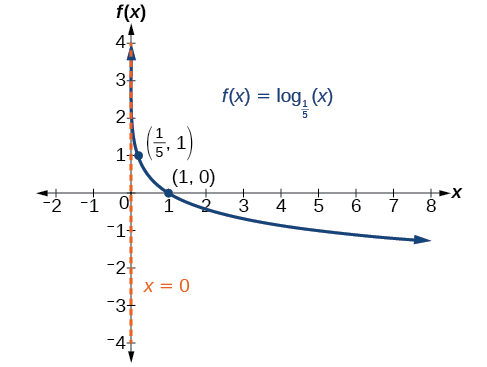# 6.4 Graphs of logarithmic functions  (Page 3/8)

 Page 3 / 8

Given a logarithmic function with the form $\text{\hspace{0.17em}}f\left(x\right)={\mathrm{log}}_{b}\left(x\right),$ graph the function.

1. Draw and label the vertical asymptote, $\text{\hspace{0.17em}}x=0.$
2. Plot the x- intercept, $\text{\hspace{0.17em}}\left(1,0\right).$
3. Plot the key point $\text{\hspace{0.17em}}\left(b,1\right).$
4. Draw a smooth curve through the points.
5. State the domain, $\text{\hspace{0.17em}}\left(0,\infty \right),$ the range, $\text{\hspace{0.17em}}\left(-\infty ,\infty \right),$ and the vertical asymptote, $\text{\hspace{0.17em}}x=0.$

## Graphing a logarithmic function with the form f ( x ) = log b ( x ).

Graph $\text{\hspace{0.17em}}f\left(x\right)={\mathrm{log}}_{5}\left(x\right).\text{\hspace{0.17em}}$ State the domain, range, and asymptote.

Before graphing, identify the behavior and key points for the graph.

• Since $\text{\hspace{0.17em}}b=5\text{\hspace{0.17em}}$ is greater than one, we know the function is increasing. The left tail of the graph will approach the vertical asymptote $\text{\hspace{0.17em}}x=0,$ and the right tail will increase slowly without bound.
• The x -intercept is $\text{\hspace{0.17em}}\left(1,0\right).$
• The key point $\text{\hspace{0.17em}}\left(5,1\right)\text{\hspace{0.17em}}$ is on the graph.
• We draw and label the asymptote, plot and label the points, and draw a smooth curve through the points (see [link] ).

The domain is $\text{\hspace{0.17em}}\left(0,\infty \right),$ the range is $\text{\hspace{0.17em}}\left(-\infty ,\infty \right),$ and the vertical asymptote is $\text{\hspace{0.17em}}x=0.$

Graph $\text{\hspace{0.17em}}f\left(x\right)={\mathrm{log}}_{\frac{1}{5}}\left(x\right).\text{\hspace{0.17em}}$ State the domain, range, and asymptote.The domain is $\text{\hspace{0.17em}}\left(0,\infty \right),$ the range is $\text{\hspace{0.17em}}\left(-\infty ,\infty \right),$ and the vertical asymptote is $\text{\hspace{0.17em}}x=0.$

## Graphing transformations of logarithmic functions

As we mentioned in the beginning of the section, transformations of logarithmic graphs behave similarly to those of other parent functions. We can shift, stretch, compress, and reflect the parent function $\text{\hspace{0.17em}}y={\mathrm{log}}_{b}\left(x\right)\text{\hspace{0.17em}}$ without loss of shape.

## Graphing a horizontal shift of f ( x ) = log b ( x )

When a constant $\text{\hspace{0.17em}}c\text{\hspace{0.17em}}$ is added to the input of the parent function $\text{\hspace{0.17em}}f\left(x\right)=lo{g}_{b}\left(x\right),$ the result is a horizontal shift     $\text{\hspace{0.17em}}c\text{\hspace{0.17em}}$ units in the opposite direction of the sign on $\text{\hspace{0.17em}}c.\text{\hspace{0.17em}}$ To visualize horizontal shifts, we can observe the general graph of the parent function $f\left(x\right)={\mathrm{log}}_{b}\left(x\right)\text{\hspace{0.17em}}$ and for $\text{\hspace{0.17em}}c>0\text{\hspace{0.17em}}$ alongside the shift left, $\text{\hspace{0.17em}}g\left(x\right)={\mathrm{log}}_{b}\left(x+c\right),$ and the shift right, $\text{\hspace{0.17em}}h\left(x\right)={\mathrm{log}}_{b}\left(x-c\right).$ See [link] .

## Horizontal shifts of the parent function y = log b ( x )

For any constant $\text{\hspace{0.17em}}c,$ the function $\text{\hspace{0.17em}}f\left(x\right)={\mathrm{log}}_{b}\left(x+c\right)$

• shifts the parent function $\text{\hspace{0.17em}}y={\mathrm{log}}_{b}\left(x\right)\text{\hspace{0.17em}}$ left $\text{\hspace{0.17em}}c\text{\hspace{0.17em}}$ units if $\text{\hspace{0.17em}}c>0.$
• shifts the parent function $\text{\hspace{0.17em}}y={\mathrm{log}}_{b}\left(x\right)\text{\hspace{0.17em}}$ right $\text{\hspace{0.17em}}c\text{\hspace{0.17em}}$ units if $\text{\hspace{0.17em}}c<0.$
• has the vertical asymptote $\text{\hspace{0.17em}}x=-c.$
• has domain $\text{\hspace{0.17em}}\left(-c,\infty \right).$
• has range $\text{\hspace{0.17em}}\left(-\infty ,\infty \right).$

Given a logarithmic function with the form $\text{\hspace{0.17em}}f\left(x\right)={\mathrm{log}}_{b}\left(x+c\right),$ graph the translation.

1. Identify the horizontal shift:
1. If $\text{\hspace{0.17em}}c>0,$ shift the graph of $\text{\hspace{0.17em}}f\left(x\right)={\mathrm{log}}_{b}\left(x\right)\text{\hspace{0.17em}}$ left $\text{\hspace{0.17em}}c\text{\hspace{0.17em}}$ units.
2. If $\text{\hspace{0.17em}}c<0,$ shift the graph of $\text{\hspace{0.17em}}f\left(x\right)={\mathrm{log}}_{b}\left(x\right)\text{\hspace{0.17em}}$ right $\text{\hspace{0.17em}}c\text{\hspace{0.17em}}$ units.
2. Draw the vertical asymptote $\text{\hspace{0.17em}}x=-c.$
3. Identify three key points from the parent function. Find new coordinates for the shifted functions by subtracting $\text{\hspace{0.17em}}c\text{\hspace{0.17em}}$ from the $\text{\hspace{0.17em}}x\text{\hspace{0.17em}}$ coordinate.
4. Label the three points.
5. The Domain is $\text{\hspace{0.17em}}\left(-c,\infty \right),$ the range is $\text{\hspace{0.17em}}\left(-\infty ,\infty \right),$ and the vertical asymptote is $\text{\hspace{0.17em}}x=-c.$

## Graphing a horizontal shift of the parent function y = log b ( x )

Sketch the horizontal shift $\text{\hspace{0.17em}}f\left(x\right)={\mathrm{log}}_{3}\left(x-2\right)\text{\hspace{0.17em}}$ alongside its parent function. Include the key points and asymptotes on the graph. State the domain, range, and asymptote.

Since the function is $\text{\hspace{0.17em}}f\left(x\right)={\mathrm{log}}_{3}\left(x-2\right),$ we notice $\text{\hspace{0.17em}}x+\left(-2\right)=x–2.$

Thus $\text{\hspace{0.17em}}c=-2,$ so $\text{\hspace{0.17em}}c<0.\text{\hspace{0.17em}}$ This means we will shift the function $\text{\hspace{0.17em}}f\left(x\right)={\mathrm{log}}_{3}\left(x\right)\text{\hspace{0.17em}}$ right 2 units.

The vertical asymptote is $\text{\hspace{0.17em}}x=-\left(-2\right)\text{\hspace{0.17em}}$ or $\text{\hspace{0.17em}}x=2.$

Consider the three key points from the parent function, $\text{\hspace{0.17em}}\left(\frac{1}{3},-1\right),$ $\left(1,0\right),$ and $\text{\hspace{0.17em}}\left(3,1\right).$

The new coordinates are found by adding 2 to the $\text{\hspace{0.17em}}x\text{\hspace{0.17em}}$ coordinates.

Label the points $\text{\hspace{0.17em}}\left(\frac{7}{3},-1\right),$ $\left(3,0\right),$ and $\text{\hspace{0.17em}}\left(5,1\right).$

The domain is $\text{\hspace{0.17em}}\left(2,\infty \right),$ the range is $\text{\hspace{0.17em}}\left(-\infty ,\infty \right),$ and the vertical asymptote is $\text{\hspace{0.17em}}x=2.$

how do I set up the problem?
what is a solution set?
Harshika
find the subring of gaussian integers?
Rofiqul
hello, I am happy to help!
Abdullahi
hi mam
Mark
find the value of 2x=32
divide by 2 on each side of the equal sign to solve for x
corri
X=16
Michael
Want to review on complex number 1.What are complex number 2.How to solve complex number problems.
Beyan
yes i wantt to review
Mark
use the y -intercept and slope to sketch the graph of the equation y=6x
how do we prove the quadratic formular
Darius
hello, if you have a question about Algebra 2. I may be able to help. I am an Algebra 2 Teacher
thank you help me with how to prove the quadratic equation
Seidu
may God blessed u for that. Please I want u to help me in sets.
Opoku
what is math number
4
Trista
x-2y+3z=-3 2x-y+z=7 -x+3y-z=6
can you teacch how to solve that🙏
Mark
Solve for the first variable in one of the equations, then substitute the result into the other equation. Point For: (6111,4111,−411)(6111,4111,-411) Equation Form: x=6111,y=4111,z=−411x=6111,y=4111,z=-411
Brenna
(61/11,41/11,−4/11)
Brenna
x=61/11 y=41/11 z=−4/11 x=61/11 y=41/11 z=-4/11
Brenna
Need help solving this problem (2/7)^-2
x+2y-z=7
Sidiki
what is the coefficient of -4×
-1
Shedrak
the operation * is x * y =x + y/ 1+(x × y) show if the operation is commutative if x × y is not equal to -1
An investment account was opened with an initial deposit of \$9,600 and earns 7.4% interest, compounded continuously. How much will the account be worth after 15 years?
lim x to infinity e^1-e^-1/log(1+x)
given eccentricity and a point find the equiationByByBy Brenna FikeBy RhodesBy OpenStaxBy Candice ButtsBy Sam LuongBy Rachel CarlisleBy RhodesBy Tod McGrathBy OpenStaxBy Jessica Collett